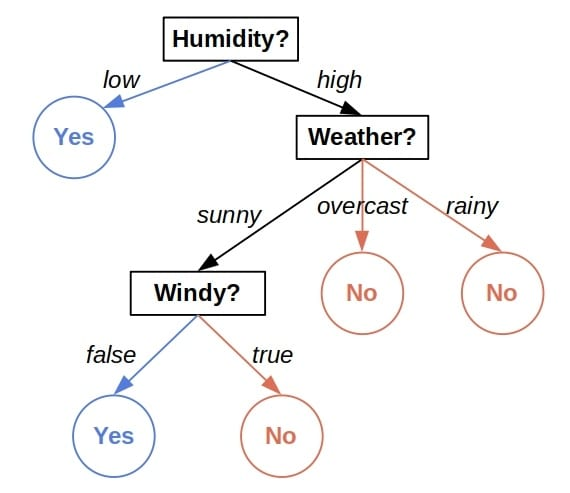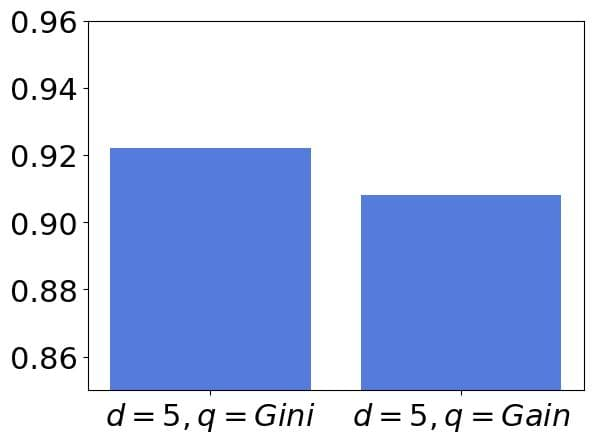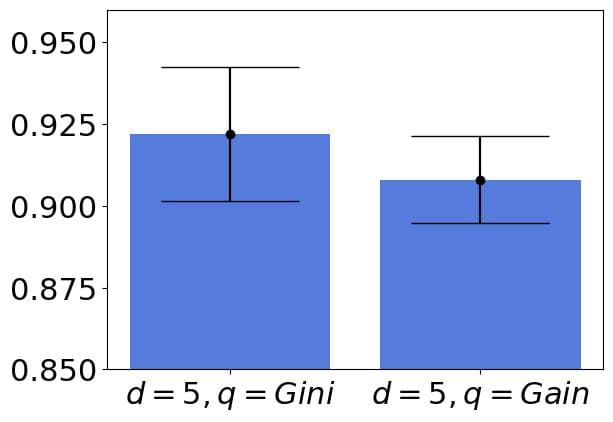## 1. Introduction

In this tutorial, we’ll explain how to perform cross-validation of decision trees. We’ll also talk about interpreting the results of cross-validation.

Although we’ll focus on decision trees, the guidelines we’ll present apply to all machine-learning models, such as Support Vector Machines or Neural Networks, to name just two.

## 2. Decision Trees

A decision tree is a plan of checks we perform on an object’s attributes to classify it. For instance, let’s take a look at the decision tree for classifying days as suitable for playing outside:Given the attributes of a day, we start at the top of the tree, inspect the feature indicated by the root and visit one of its children depending on the feature’s value. Then, we repeat the process until we reach a leaf node and read the decision.

## 3. Cross-Validation

Two kinds of parameters characterize a decision tree: those we learn by fitting the tree and those we set before the training. The latter ones are, for example, the tree’s maximal depth, the function which measures the quality of a split, and many others.

They also go by the name of hyper-parameters, and their choice can significantly affect the performance of the decision tree. So, a natural question is how to set the hyper-parameters to increase the performance of the resulting tree as much as possible. To do that, we usually conduct cross-validation.

First, we need to decide which hyper-parameters we’ll tune. The thing is that there are a lot of them, and rigorously testing each combination of values can take too much time. For instance, let’s say that we decided to work with two hyper-parameters:

•: the maximal depth of the tree.
•: the function to measure the quality of a split.

Next, we identify which hyper-parameter values we want to test. For example,, and, whereis the information gain, andis the Gini impurity. That way, we get a grid ofcombinations:We’ll train and validate a tree using every combination from the grid.

### 3.2. The Usual Cross-Validation

The usual way to cross-validate a tree is as follows. We split the data into the training and test sets. Then, we split the training data intofolds:(, or more, depending on our computational capacities). When dealing with classification problems, the best practice is to keep the ratio of different classes in each fold approximately the same as in the entire dataset.

Afterward, we iterate over the folds. In the-th pass, we use all the folds butto train a tree for each combination in the grid, validating the fitted tree on the reserved fold. That way, we gettrees andvalidation scores for each grid combination.

### 3.3. The Results

If there arecombinations in the grid, and we split the training set intofolds, we’ll have antable of validation scores. Each score results from testing the tree on the fold we didn’t use to train it. Visually, it’s a two-dimensional matrix:The valueis the performance score we get by training the tree on folds other thanusing thecombination in the hyper-parameter grid and evaluating it on. For example, if we measure accuracy, we may get the results like this with:### 3.4. Selecting the Best Combination

Finally, we set the hyper-parameters to the combination which gave the best tree. Usually, we go with the settings having the best mean value. However, means can mislead us if we don’t account for the variability of scores. For instance, the mean accuracy forin the above table is, while the mean accuracy foris:As the accuracy difference ofcan be substantial in the domain where we will use the tree, we could conclude that the combinationallows for training more accurate trees. But, if we calculate the standard deviations and add the margin errors, we’ll see that the intervals overlap:In such cases, we should choose the computationally less intensive settings or those that lead to simpler models. For instance, if we considered only the depth hyper-parameter and the intervals forandoverlapped, we’d go with. The reason is that the shallower trees work faster and are easier to understand

Alternatively, we could calculate additional performance scores to break ties or look for the combination(s) in the Pareto front of scores. Another option is to run a statistical test on the scores to find which combination provides the most accurate trees.

However, we should keep in mind that even if we found a statistical difference between two combinations’ scores, that wouldn’t mean that the trees trained under one hyper-parameter setting are necessarily better than those trained under the other combination. For instance, we may consider the trees whose mean accuracy scores are withinthe same even if the error bars overlap.

### 3.5. The Nested Cross-Validation

That’s how cross-validation is usually done in practice. However, the approach has a shortcoming. Since we first split the data into the training and test sets and then cross-validate the tree using the folds of the training set, our results are conditional on the main train/test split. If our dataset is small, the final tree’s performance on the test set can be an imprecise estimate of its actual performance.

Nested cross-validation addresses this issue by iterating over data splits as well. Namely, we split the data into the training and test foldstimes. Further, we perform the cross-validation as described above for each of thesplits.

That way, we eliminate the effect of data splits (if any) and avoid sampling bias. But, the main disadvantage is that we dotimes more work, which we may not be able to afford.

### 3.6. Example

Here’s an example of the result matrix of the nested cross-validation withouter andinner splits:Now, we havescores per combination, which better estimates the mean values.

## 4. Interpreting the Results of Cross-Validation

Since each fit can give a different tree, it may be hard to see the meaning of averaged validation scores. Thevalidation scores we get for a combination in a grid are a sample of the performance scores of all the trees we can get by training tree models using that particular train set under that particular combination of the hyper-parameter valuesTheir average estimates the expected performance. So, the mean values we get don’t refer to a specific tree. Instead, they represent the expected performance of a family of trees, characterized by the settings of the hyper-parameters and the initial train/test split.

When it comes to nested cross-validation, we get the scores for different train/test splits. So, the average value for a combination estimates the expected performance of a tree trained for that particular problem under those particular hyper-parameter settings, regardless of how we split the data into train and test sets.

## 5. Conclusion

In this article, we talked about cross-validating decision trees. We described non-nested and nested cross-validation procedures. Finally, we showed the correct way of interpreting the cross-validation results.